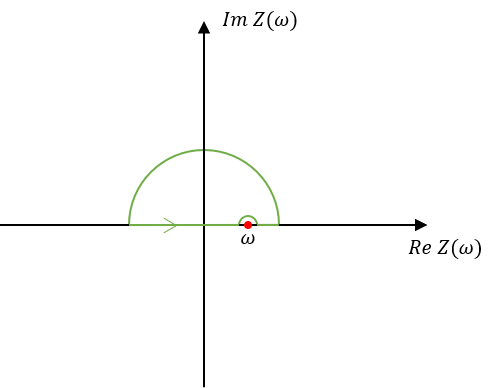0%

$$Z'(\omega) = \frac{1}{\pi} P \int^{\infty}_{-\infty} \frac{Z''(\omega')}{\omega'-\omega} \text{d}\omega'$$
$$Z''(\omega) = -\frac{1}{\pi} P \int^{\infty}_{-\infty} \frac{Z'(\omega')}{\omega'-\omega} \text{d}\omega'$$

# Kramers–Kronig关系成立的四个条件

• 因果性条件：物理体系只对所施加扰动进行响应。对信噪比有要求。
• 线性条件：物理体系的响应与施加扰动间为线性关系。施加扰动不可过大——在小扰动范围内，电压与电流近乎线性关系。
• 稳定性条件：对体系停止扰动后，体系能回到受扰动前的状态。施加扰动不可过大，从而使体系不转移到另一个状态。
• 有限性条件：随频率变化的物理量（阻抗）在全频率范围内（包括零和无穷大）都是有限值。部分RC电路不满足有限性条件，因此也不存在Kramers–Kronig关系。

# Kramers–Kronig关系的证明# 参考资料

 曹楚南. 电化学阻抗谱导论[M]. 科学出版社, 2002.
 https://en.wikipedia.org/wiki/Kramers–Kronig_relations.